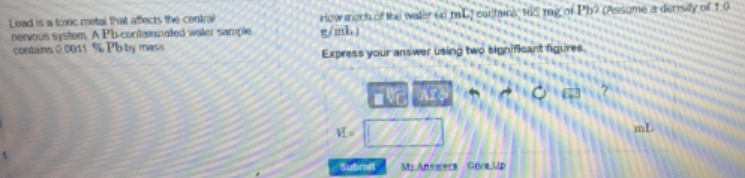# Problem: Load is a basic metal that affects the central nervous system. A Pb-water sample contains 0.0011 % Pb by mass.How much of the water (in mL) contains 1.65 mg of Pb? (Assume a density of 1.0 g/mL) Express your answer using two significant figures.

###### FREE Expert Solution
80% (16 ratings)###### Problem Details

Load is a basic metal that affects the central nervous system. A Pb-water sample contains 0.0011 % Pb by mass.

How much of the water (in mL) contains 1.65 mg of Pb? (Assume a density of 1.0 g/mL) Express your answer using two significant figures.